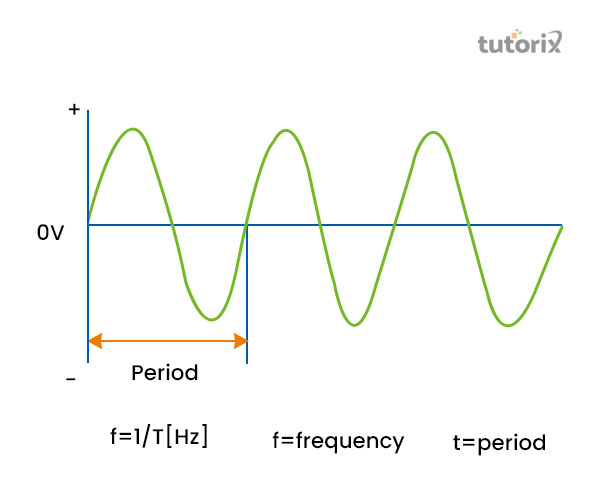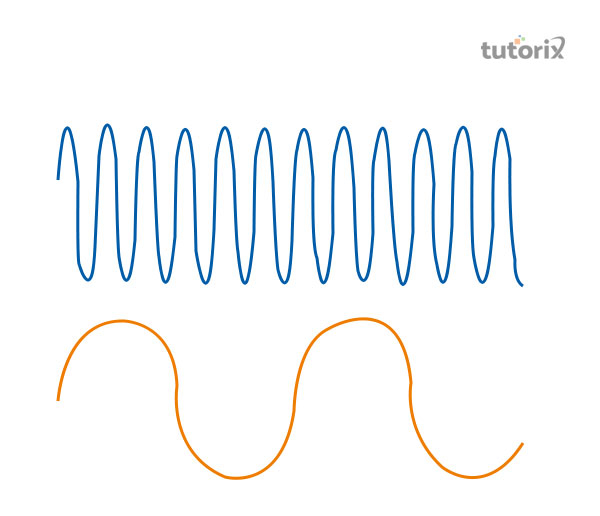# Relation Between Frequency and Velocity

## Introduction

In order to get introduced to the relationship between velocity and frequency, it is necessary to understand the concept of waves and the characteristics displayed by waves.

A wave can be defined as the disturbances that are noticed within a medium carrying energy. However, within the medium, there lies no net movement of the particles. These waves take several forms and the aspect of frequency and velocity better defines these.

## Definition of Frequency

The wave may take several forms, such as the shape of "elastic deformation", differences between the aspects of pressure, the intensity of magnets and electricity.

Furthermore, the way can also be displayed in the form of variation in the "electric potentials" and temperatures. However, through waves, energy is being transferred, and that involves repetitive as well as periodic motions and does not support the occurrences of net movement of the particles within the medium (Yuan et al. 2019).

The descriptions define the term wavelength to be the distance that lies between the "identical parts" within the wave. The term "amplitude" is referred to as the displacement that occurs at the maximum level from the wave's neutral positions.

Frequency is defined as the total number of waves that pass through "one point" within a "unit of time". The frequency is referred to as the measurements that are fundamental to the display of waves. However, the unit of "Hertz (Hz) determines the frequency of the wave (Tlili et al. 2018). It can also be stated to determine the number of vibrations of cycles that are conducted within the "unit time", by the concerned body within its periodic motions. In the figure given below, the frequency is stated with the net number of waves passed under a specific point and under a specific time.Figure 1: Frequency

## Factors associated frequency

Frequency is stated as the nature of the source that supports evaluating the nature of the characteristic of waves. In addition to these, much needs to be noted about the factors that tend to influence the nature of frequency. The factor responsible for the change in frequency is the property of the materials with which the object is made, as this affects the speed associated with waves (Brainkart.com, 2022).

However, the length of the material is also a factor that influences the frequency, as it affects the "wavelength" of the respective waveform.

## Types of frequency

Frequency can be of two types noticed in the field of physics in determining the exact measurements of several types of vibrations that may include oscillations, waves and rotations. However, these passing vibrations need to be under "unit time" (Qrg.northwestern.edu, 2022).

• The completion of this cyclic process occurs in two types, one is "high frequency" and another one is "low frequency" waves.
• "High frequency (HF)" is defined as the range of electromagnetic waves ranging between "3 to 30 MHz (megahertz)" (Fluke.com, 2022).
• On the other hand, "low frequency (LF) waves" can be defined as, those that range from, "30 to 300 kHz or (kilohertz)".Figure 2: Types of Frequency

## Definition of velocity

Velocity is stated as the total distance that is travelled by a point and therefore, it is denoted as the prime indicator of the position of the object. In addition to these, the nature of rapidity is also determined by the notion of velocity (Thoughtco.com, 2022).

In simple terms, it is the distance travelled by an object within a specific period. However, the movement needs to be conducted in a particular direction.

For example, the movement of a wave with a proper speed within a particular period of time causes a movement in a defined direction, which can be stated as the velocity of the wave (Techtarget.com, 2022). However, the aspect of velocity is defined as measured by the unit of "meter per second''. Therefore, we can understand a lot about the nature of the wave from its velocity.

## Characteristics of velocity

Velocity is known to be a vector quantity that provides both the notions of magnitude and direction of the waveform (Hioki.com, 2022).

The "instantaneous speed" is denoted by the magnitude of the "vector velocity" and the direction occurs in the same direction in which the wave is moving.

## Relation between velocity and frequency

The relationship between the notion of velocity and frequency is directly proportional, this is determined that if there is an increase in the notion of frequency, then the velocity of the concerned waveform also increases (Circuitglobe.com, 2022).

However, one should keep in mind that the wavelength needs to be constant for the above condition.

### Formula representing frequency to the velocity of the wave

"Velocity" of the wave is measured by, frequency multiplied by the wavelength, that means, "V = f * λ". However, if the wavelength or "λ" remains constant then, velocity is doubled if the frequency is doubled and so on.

## Conclusion

In this tutorial, an intensive idea has been provided that concerns the aspects of velocity and frequency, which are very essential in studying the nature of waves, and oscillations. The waves can also be in cyclic motions as they represent a repetitive motion. The nature of a wave is determined by frequency in order to evaluate how fast or slow the wave is moving in a particular direction. However, velocity determines the intensity of waves.

Q1. How is velocity related to the frequency of the wave?

Frequency and velocity are "directly proportional" to each other while wavelength remains constant.

Q2. How is the aspect of the "time period" evaluated?

The time period is evaluated by catering to the relation between "velocity" and "frequency". The formula is detonated by "T = 1/f", where, "f" denotes the frequency and "t" is "time period".

Q3. How is wavelength expressed?

"Wavelength" of the wave is measured and expressed as metre, and metre is denoted by "m".

## References

### Journals

Tlili, S., Gauquelin, E., Li, B., Cardoso, O., Ladoux, B., Delanoë-Ayari, H., & Graner, F. (2018). Collective cell migration without proliferation: density determines cell velocity and wave velocity. Royal Society open science, 5(5), 172421. Retrieved from: https://royalsocietypublishing.org/doi/abs/10.1098/rsos.172421

Yuan, S., Wang, S., Luo, Y., Wei, W., & Wang, G. (2019). Impedance inversion by using the low-frequency full-waveform inversion result as an a priori model. Geophysics, 84(2), R149-R164. Retrieved from: https://library.seg.org

Websites

Circuitglobe.com, (2022), what is the frequency? , Retrieved from: https://circuitglobe.com [Retrieved on, 11th June 2022]

Fluke.com, (2022), what is frequency? , Retrieved from: https://www.fluke.com [Retrieved on, 11th June 2022]

Hioki.com, (2022), what is frequency definition, Retrieved from: https://www.hioki.com [Retrieved on, 11th June 2022]

Qrg.northwestern.edu, (2022), what is frequency? , Retrieved from: https://www.qrg.northwestern.edu [Retrieved on, 11th June 2022]